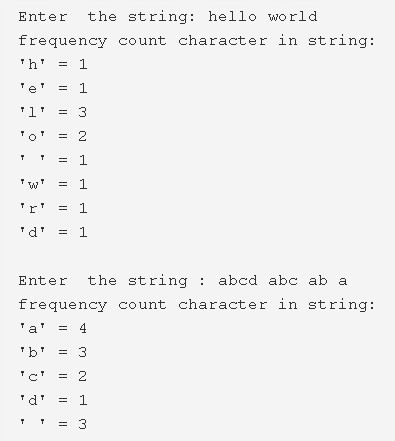# C Program To Count Frequency Of Each Character In String | C Programs

C program to count the frequency of each character in a string – In this article, we will describe the numerous means to count the frequency of each character in a string in C programming.

Suitable examples and sample programs have also been added so that you can understand the whole thing very clearly. The compiler has also been added with which you can execute it yourself.

The means used in this piece are as follows:

• Using Standard Method
• Using Function

A string is nothing but an array of characters. The value of a string is determined by the terminating character. Its value is considered to be 0.As you can see, firstly, you need to enter the particular string for which you want to check the characteristics for.

The string entered in the first example, as the image uploaded above suggests, is as follows:

“hello world”

It is clearly evident that these are the characters used in this string:

• ‘h’ = 1
• ‘e’ = 1
• ‘l’ = 3
• ‘o’ = 2
• ‘ ‘ = 1
• ‘w’ = 1
• ‘r’ = 1
• ‘d’ = 1

Thus, the methods to find out the same in C programming are as follows:

## Using Standard Method

1. Read the string entered by the user and store the string into the variable ‘s’ using gets(s).

2) The for loop iterates through the string with the structure for(j=0;s[i];j++) until the last character of the string becomes null.

a) Initialize the j value to the variable n.j indicates the length of the string.

3) Count=0.Now count the frequency of each character in the string,

a) The outer for loop iterates through the string with the structure for(i=0;i<n;i++),for each iteration it selects one element s[i].

b) The inner for loop compares the s[i] with remaining elements of the string using for loop with the struct for(j=i+1;j<n;j++).If it matches then increase the count value.

c) Print the count value which is the frequency of the selected element s[i].

Output:

### Using Function

1. The function stringlength(char *s) returns the length of the string.

2) The main() function calls the function printfrequencyofcharacters(char *s) by passing the string as an argument to the function.

3) The function printfrequencyofcharacters(char *s) prints the frequency of each character of the string.

The outer for loop iterates through the string with the structure for(i=0;i<n;i++).

a) For each iteration of the outer for loop, the inner for loop will compare the s[i] with the remaining characters of the string.

b) If s[i] matches then increase the count value and print the count value of s[i] which is the frequency of s[i].

Output:

x

## C Program To Print Number Of Days In A Month | Java Tutoring

C program to input the month number and print the number of days in that ...